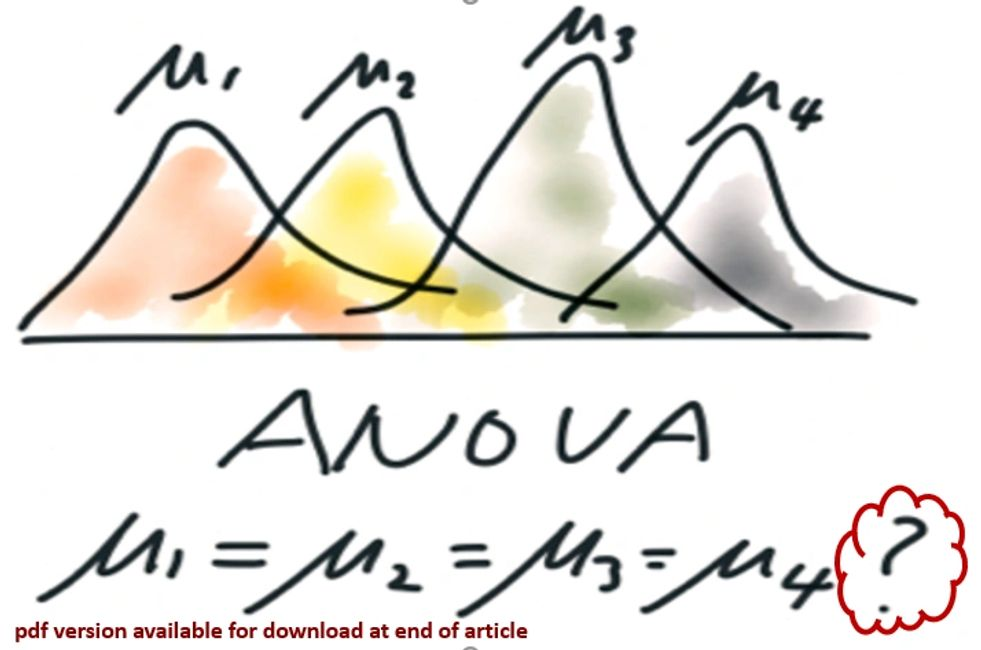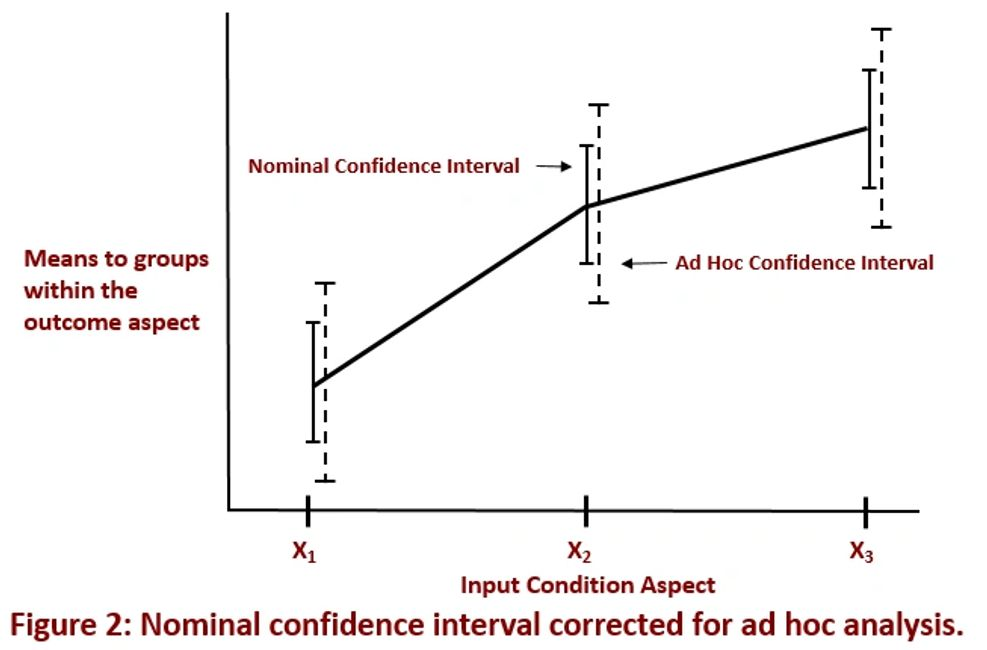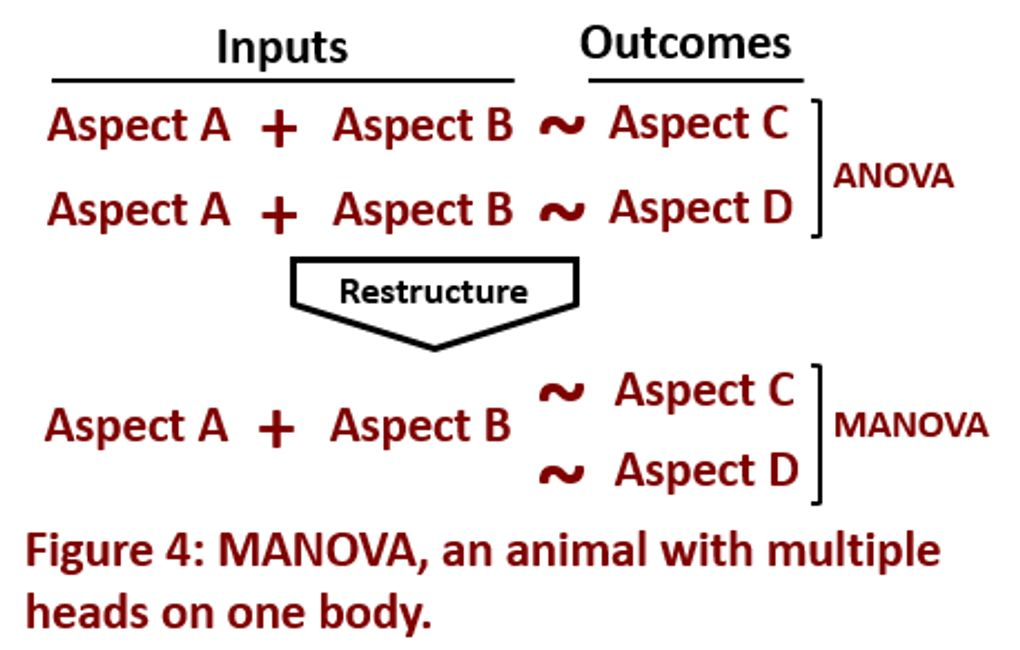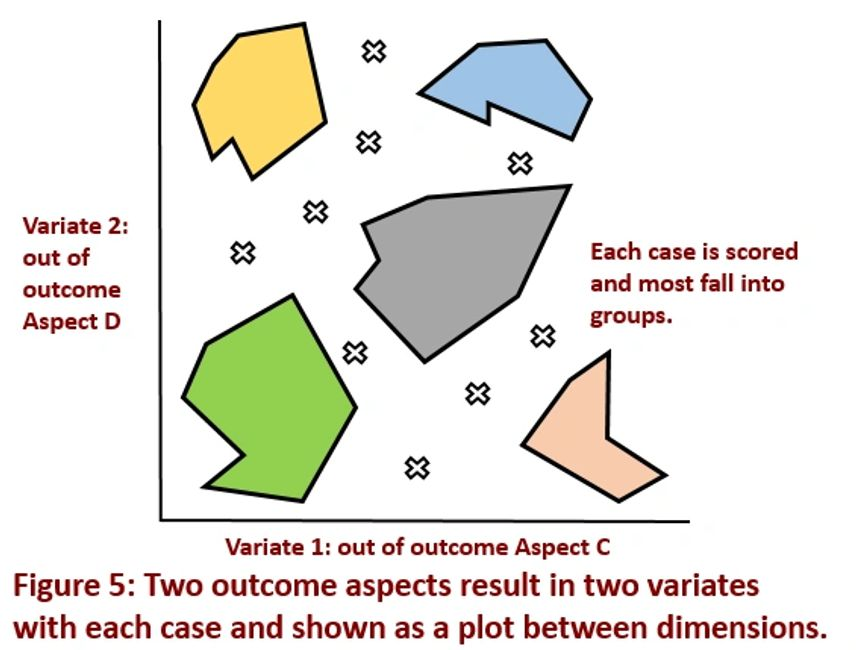New-Age Five Questions >> Know that Improvements Work by Asking Difference QuestionsBy Richard G. Lamb, PE, CPA; Analytics4Strategy.com

Driving operational processes toward excellence depends upon being able to distinguish between true and false differences in a process’s outcome aspects after making improvements. We must be able to expect that the improvements made to process elements will have a significant impact and then be able to confirm that there is an impact.

This article is a Plain-English explanation of difference questioning. Difference questioning is one of five core types of questioning—relationship, difference, time series, duration and apparency—all which play together to augment a team’s ability to reach for operational excellence.

The article will also explain the tools that teams would use to ask and answer difference questions. They are two-mean comparison, one-way and multi-way ANOVA, ANCOVA, repeated-measures and mixed ANOVA, and MANOVA. Rather than technical, the tools are presented with respect to the kinds of questions we would ask and answer with them.

However, the explanation we all want in the end is how to conduct the explained exploration. The article, “DMAIC Done the New-Age Way,” explains how the apparency questioning of this article, along with the four other core types of questioning are woven into the stages to define, measure, analyze, improve and control processes. Although presented in the context of DMAIC, the explanation is universal to any problem solving sequence.

What We Are Really Doing

What we are actually and simply doing is searching for all true differences between the means of groups in an outcome aspect of an operations process. If we work through the DMAIC stages without teasing them out, the organization will suffer the worst of all wastes and breakdowns—building and managing the right solutions for the wrong problems.

Figure 1 shows the nature of the beast. The big glob represents an outcome aspect without regard for one or more input aspects with two or more conditions (e.g., before and after). However, when we relate the glob to the conditions of input aspects, it subdivides into groups around each group. Each group has its own mean and shape, and explains some part of the glob.There are no limits to the input aspects and conditions we can associate with the glob. For example, the figure could be a single input aspect of four conditions or two aspects with two conditions. If we had two input aspects of three conditions each, the figure would show nine groups.

However, the overarching challenge is always to determine which of the groups are truly different, rather than only numerically different. To speak statistics, which are, and are not, from different populations hidden in the glob? The answer will set us free!

Seek and You Shall Find Truth

When I began studying statistics, a whole new world opened for me upon learning that numerically different outcomes are not necessarily different outcomes. Furthermore, contrary to my instincts, I learned that determining the truth is more complex than merely comparing all pairs of groups using the basic-most statistical method (t-test) to compare two means. The problem is what is called “familywise error.”

To demonstrate, imagine that Figure 1 is an ANOVA model of a single input aspect of four conditions for which our confidence is 95 percent that at least 2 outcome aspect groups out of 6 have statistically different means. However, when we try to determine exactly which pairs are true differences, we can only be 74 percent (0.956) confident that we will get it right.

The solution lays in two quantitative methods. In statistics-speak, they are called ad hoc and contrasts.

The “ad hoc” method explores pairs in a table of comparisons, but with no particular comparison in mind. The method attempts to correct for familywise error rather than step around it.

A correction is applied to the level of significance at each pair in order to reflect the familywise significance. For example, we may use a 99 percent significance for each pair as a way to arrive at a conclusion that reflects 95 percent overall.

It is obvious from Figure 2 that the ad hoc method introduces conservatism to the conclusions: however, we must still be cautious. While avoiding false positives (e.g., telling a man he is pregnant) to we may fall into false negatives (e.g., telling an almost full-term pregnant woman she is not).Figure 2 shows the concept for a three condition input aspect. A gap between respective confidence interval bars indicate a true difference. The nominal case shows there to be two differences out of three. However, the corrections of the ad hoc method show only one difference.

Contrasts are coded into ANOVA models to answer specific questions of comparison rather than merely compare paired groups. Contrasts are the best of the two methods. This is because the comparisons of one or more groups of means are made by comparing the relative variance each explains in the total variance. Accordingly, the confidences are unique to each contrast rather than familywise.

Contrasts are built for each input aspect. We may start by asking if group X1 is different than the average of X2 and X3 as a group. Thence, we will ask if there is a difference between X2 and X3.

We are free to build any set of contrasts as necessary to explore all differences of interest to us. They will ask more sophisticated questions than merely pairings. However, we can test all pairs with contrasts designed to do so. We can also build contrasts to determine if there are linear and higher order trends across the groups.

But what about models with two or more aspects of two or more conditions? What if we wanted to integrate two or more input aspects? For example, three input aspects with three, four and three conditions respectively.

The trick is to create a new input aspect in the dataset. It is the 36 combinations (32X41) of conditions. We then build a model with the newly created aspect as a single input aspect. Thence, we can use both methods to explore the differences.

Independent, Repeated or Mixed Design?

There is a final structural question to settle before jumping into the power tools of difference. Are we dealing with an independent or repeated design? ANOVA models are designed as one or the other, or as a mixture.

Let’s clarify with a model of one input aspect with two conditions—before and after an improvement. An independent design randomly assigns each case in the operational dataset to only one of the two conditions. A repeated design assigns each case to both conditions. Another way to say it is that one case contributes to all mean groups.

The principle extends to models with two or more input condition aspects. However, we may treat some aspects as independent and the others as repeated—mixed design. Consequently, each case will contribute to some mean groups, but not all.

The data from operational processes allow us to explore for difference with any of the three designs—independent, repeated and mixed. This is because data is captured generally by the enterprise’s operating and ERP systems as needed to run the business as a system of interrelated operations. It is not in their design to conduct data analytics beyond trivial.

Instead, the issue is to find a model which is the best possible fit to the process data. This is because there is a serious penalty to the enterprise if we build a model of independence when a repeated or mixed model is a better fit. The way to avoid disasters is to build variations and judge them by their relative fit.

The Power Tools of Differences

It is easy to get swept up in the elegance of the statistical difference tools while missing the power of questioning they give us. This section will name and summarize the tools in the context of the questions they allow us to ask and answer. They are presented in the order of two-mean comparison, one- and multi-way ANOVA, ANCOVA, repeated-measures and mixed ANOVA, and MANOVA.

Two-mean comparison (t-test): We will start at what is not an ANOVA model, but answers the same question. Is there a difference? The two-mean comparison comes into play when there is a single input aspect with only two conditions and, therefore, only two outcome groups.

The two-mean comparison analytic is a simple statistical calculation. With what is called the t-test, the method combines the variances of the respective means to judge the gap between the two means. The calculation differs as the cases are of an independent or repeated design (i.e., each case contributes to one or both outcome groups).

One-way and multi-way (aka, factorial) ANOVA: Enter the ANOVA models starting with one- and multi-way. The overarching question is the same, but requires stronger quantitative tools than a two-means comparison. This is because we are now evaluating differences among three or more output groups.

With respect to independent or repeated design, this is the independent case. Changing the independent model to repeated design will be broached later as “repeated-measures” ANOVA.

A one-way ANOVA applies to groups from a single input aspect with three or more conditions—looks like Figure 2. A multi-way ANOVA has two or more input aspects, each with two or more conditions—looks like Figure 3.With ANOVA our first question is, “do one or more of the input aspects actually create outcome groups with means that are significantly different from the output aspect’s mean?” If there are two or more input aspects, the second question is, “do any of the input aspects interact and which ones?”

Figure 3 shows the case of two input aspects with three conditions. Because there are only two input aspects, we can visually answer both questions, but cautiously. What is shown “may” be differences with the grand mean and the non-parallel cases “may” be interactions. For both questions, the ANOVA model gives us statistical confidence beyond what is visualizable.

The final question is, “which means of the paired and more complex contrast groups are actionably different?” There are two vantages to work from.

The first vantage evaluates the model’s input aspects individually as if each were the case of Figure 2. The groups out of each input aspect are compared pairwise with the ad hoc method. They are also evaluated per the contrasts we have coded for each input aspect.

To find differences hidden within the integration of two or more input aspects and their respective conditions, the second vantage combines the conditions of all input aspects as permutations. Thence, we would conduct the ad hoc and contrast analytics on the single input aspect.

Interestingly, we are reverting from multi- to one-way ANOVA. We are moving from visualizing the groups formatted akin to Figure 3 to visualizing the groups formatted akin to Figure 2.

We will typically work from both vantages. We can create additional vantages through multi-way models which combine fewer than all input aspects. As we work from the vantages, we will likely reach back to the source datasets to restructure (e.g., collapse or create hybrid conditions) the original input aspect conditions based on what we are discovering.

Analysis of covariance (ANCOVA): Our question overall is which of the conditions from input condition aspects point to significantly different populations in the outcome aspect. To more confidently make the distinction, we now explore whether to extend the ANOVA model upon another question.

The question is, “are there other process aspects which are related to the outcome aspect, but are random rather than choices between input aspect conditions?” The question seeks what is called “covariates.” They are typically numeric rather than conditions.

At every chance, the article has avoided statistics-speak and, instead, focus on what we are doing. Now we need a bit of it. It is that ANOVA is an acronym for analysis of variance based on a simple concept.

For each input condition aspect, ANOVA analyzes how much of the total variance of the output aspect (the glob of Figure 1) is explained by each input aspect compared to what is not explained by any input aspect. The conclusions of significance made by ANOVA are based on the ratio of explained to unexplained.

We needed the statistics-speak to explain one of the two primary reasons to work with covariates. They explain some part of the otherwise unexplained variance in the model. This makes explained variances more prominent vis-a-vis the reduced remaining unexplained variance. Added to that is that if a covariate influences the outcome aspect, including them removes their bias from the mean groups.

The sequence of questions is the same as for one- and multi-way ANOVA—significant effects by input aspects, interactions between aspects and mean groups. However, we are now answering them after adjusting for, in statistic speak, the unique correlation between the covariates and the output aspect. Imagine the groups of Figures 2 and 3 as variously shifted vertically and, therefore, telling us a different story.

Repeated-measures and mixed-design ANOVA: Now enter repeated-measures ANOVA. Recall that a repeated-measures ANOVA is one in which each case will be measured at every condition of the input aspects. As the name suggests, the mixed-design ANOVA will treat some input condition aspects as repeated and others as independent.

In contrast to one- and multi-way ANOVA, the explained variance in a repeated-measures design will have two components of variance. One is the explained variance “within” cases after removing the peculiarities of each case. The other is the explained variance “between” cases.

As mentioned previously, we most precisely find wastes and breakdowns by making the appropriate design choice for each input aspect. We do that by finding the model design that best fits the data. This is done by progressing through iterations of adding one aspect at a time, making one choice at a time and then comparing each fit to its predecessor model. Recall that the generalized nature of operations data allow us to construct variations.

Ultimately, we use the fitted model to explore the differences between mean groups of the outcome aspect. We will do that just as before with the ad hoc comparisons and constructed contrasts.

Multivariate ANOVA (MANOVA): Enter MANOVA as the means to distinguish outcomes which cannot otherwise be seen through the lens of the previous ANOVA structures. Now we look for differences by investigating the relationship between two or more outcome aspects in a single model.

MANOVA is hard to visualize because it is so different from our ingrained idea of an “equation.” To jump the hurdle, Figure 4 shows the difference between ANOVA and MANOVA.

As always, we have a body of input aspects. Until now, we have presented what is shown in the upper part of the figure—one body, one head. Now we have moved to the lower part of the figure—one body, two or more heads.

Just as in all of the previous “univariate” ANOVA models, we first determine if there is something going on and, if so, which input aspects significantly influence outcomes. If the model is positive, our next task is still to find the true differences. Both are answered with what is called “eigenvectors and eigenvalues.”

Eigenvalues are used to compare explained and unexplained variance in the model to determine if something is going on and which inputs aspect have effect. Eigenvectors, through what is called “discriminate function analysis,” are used to answer the question of difference.To tease out the differences, the analysis converts each outcome aspect to a dimension, called a “variate.” In turn, dimensional functions (akin to a regression with its eigenvectors as coefficients) convert each data case to a score along the respective dimensions.

Discriminate function analysis is actually machine learning. This is because MANOVA works backward from the multiple output aspects to learn how to score each case along the respective factors. This is called directed learning because we give the model the outcome aspects and ask it to learn how to get us there.

Figure 5 shows the concept of what is learned in a two-outcome case. The axes represent the variates to the respective outcome aspects C and D of Figure 4. Imagine, as shown, that most of the individual plot points tend to fall into one of the bordered groups. It is up to the project team to give meaning to the groups.However, there is a further opportunity to explore for insight. The team can classify the cases in its datasets by names based upon the groups. In other words, we are creating a new input condition aspect that does not exist in the enterprise’s operating systems. With it, we can revert to the ANOVA models. The models can be based solely on the new aspect. Alternately, we can combine the new input aspect with other input aspects and covariates.

Sources for self-directed learning: Discovering Statistics Using R, Field and Miles, 2012 | Multilevel Modeling Using R, Holmes, 2014 | Machine Learning with R, Lantz, 2015 | ggplot2, Elegant Graphics for Data Analysis, Wickham, 2016 | Introductory Time Series with R, Cowpertwait and Metcalfe, 2009 | Event History Analytics with R, Bostrom, 2012 | Package “tsoutliers,” Javier López-de-Lacalle, 2017